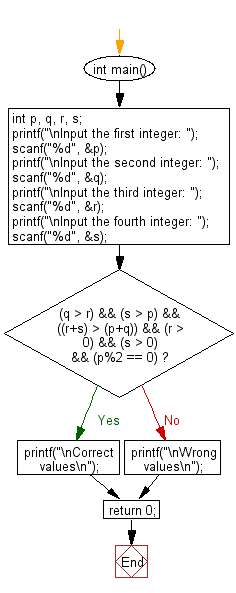﻿ C Program: Accepts 4 integers and check various condition - w3resource

# C Exercises: Accepts 4 integers and check various condition

## C Basic Declarations and Expressions: Exercise-19 with Solution

Write a C program that accepts 4 integers p, q, r, s from the user where q, r and s are positive and p is even. If q is greater than r and s is greater than p and if the sum of r and s is greater than the sum of p and q print "Correct values", otherwise print "Wrong values".

Test Data :
Input the second integer: 35
Input the third integer: 15
Input the fourth integer: 46
Expected Output:
Wrong values

C Code:

``````#include <stdio.h>
int main() {
int p, q, r, s; // Declare variables for four integers

// Prompt user for the first integer and store in 'p'
printf("\nInput the first integer: ");
scanf("%d", &p);

// Prompt user for the second integer and store in 'q'
printf("\nInput the second integer: ");
scanf("%d", &q);

// Prompt user for the third integer and store in 'r'
printf("\nInput the third integer: ");
scanf("%d", &r);

// Prompt user for the fourth integer and store in 's'
printf("\nInput the fourth integer: ");
scanf("%d", &s);

// Check conditions for correctness
if((q > r) && (s > p) && ((r+s) > (p+q)) && (r > 0) && (s > 0) && (p%2 == 0))
{
printf("\nCorrect values\n");
}
else {
printf("\nWrong values\n");
}

return 0;
}

``````

Flowchart:Sample Output:

```Input the first integer: 25

Input the second integer: 35

Input the third integer: 15

Input the fourth integer: 46

Wrong values
```

C Programming Code Editor:

What is the difficulty level of this exercise?

Test your Programming skills with w3resource's quiz.

﻿